Well Hydraulics

# Well Hydraulics - Notes | Study Civil Engineering SSC JE (Technical) - Civil Engineering (CE)

 1 Crore+ students have signed up on EduRev. Have you?

Chapter 4:
Well Hydraulics

SPECIFIC YIELD (Sy:
The specific yield of an unconfined aquifer is the ratio of volume of water which will flow under saturated condition due to gravity effects to the total volume of aquifer (V).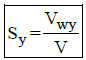where, VWY = Volume of water yielded under gravity effect and V = Total volume of water.

SPECIFIC RETENTION:
The specific retention of an unconfined aquifer is the ration of volume of water retained against gravity effect to the total volume of aquifer (V)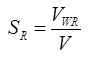where Vwr =  Volume of water retained under gravity effect

Remember:-
S+SR=n where, n = porostiy

COEFFICIENT OF TRANSMISSIBILITY:
T=kH
where,
H = Thickness
K = Coefficient of permeability

UNCONFINED AQUIFER: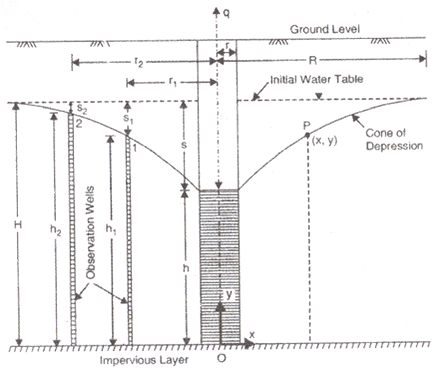(a) Theims Theory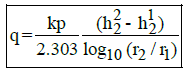Where,
q = Rate of flow in m3/s
h1 = Height of water table of 1st observation well
h2 = Height of water table of 2nd observation well
s1 = Drawdown of 1st test well
s2 = Drawdown of 2nd test well.
h1 + s1 = h+ s2
S1 = Drawdown of 1st test well
S2 = Drawdown of 2nd test well
r1 and r2 are radius of 1st and 2nd observation wells respectively.

(b) Dupits Theory: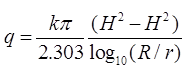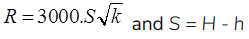where, S = Drawdown in the well
K = Permeability coefficient in m/s.
R = Radius of influence in ‘m’
150m ≤ R ≤ 300m
r = Radius of test well in ‘m’.
Results of dupits theory are not accurate because ‘R’ is based on empirical relation.

CONFINED AQUIFER: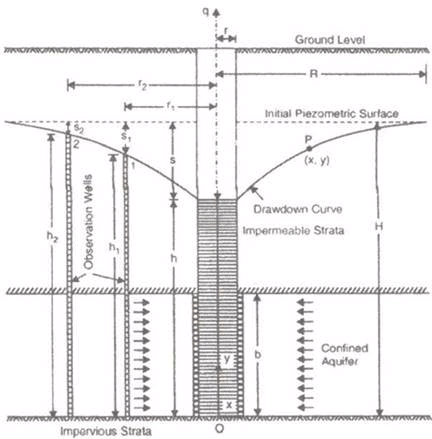(i) Theims theory: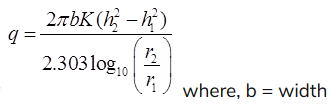(ii) Dupits theory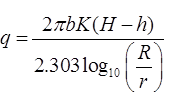SPHERICAL FLOW THROUGH WELL
qs=k2πrs, R = Radius of well
S = Drawdown
qs = Rate of flow through spherical well in m3/s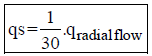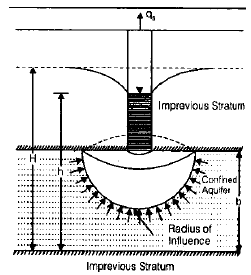PUMPING-IN-TEST
(a) Open end test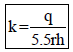where, r = radius of pipe, h = head of water above the base of pipe, it may include gravity head and pressure head.

(b) Tacker test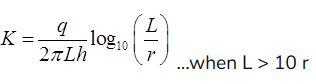where, L = Length of perforated section of pipe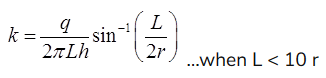OPEN WELL (RCUPERATION TEST):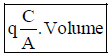where,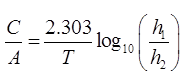Volume = A. H
A = Area of well
C/A = specific yield or specific Capacity of an open well.
T = Time in ‘sec’
h1 = Position of water table of t = 0
h2 = position of water table of t = T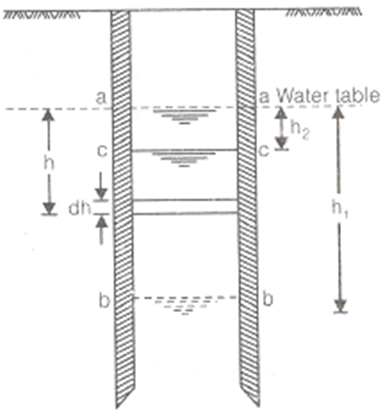VALUES OF PERMEABILITY:

 SOIL K (cm/sec) Degree of permeability 1. Coarse Gravel >1 High 2. Fine gravel-Fine sand 1 to 10-2 medium 3. Silt-Sand admixtures,loose silts, rock flour and loess 10- 2 to 10-4 Low 4. Dense slit, clay siltadmixutures,non-homogenous clays 10-4 to 10-6 Very low 5. Homogenous clays < 10-6 Impervious
The document Well Hydraulics - Notes | Study Civil Engineering SSC JE (Technical) - Civil Engineering (CE) is a part of the Civil Engineering (CE) Course Civil Engineering SSC JE (Technical).
All you need of Civil Engineering (CE) at this link: Civil Engineering (CE)

## Civil Engineering SSC JE (Technical)

2 videos|122 docs|50 tests
 Use Code STAYHOME200 and get INR 200 additional OFF

## Civil Engineering SSC JE (Technical)

2 videos|122 docs|50 tests

Track your progress, build streaks, highlight & save important lessons and more!

,

,

,

,

,

,

,

,

,

,

,

,

,

,

,

,

,

,

,

,

,

;